Anúncio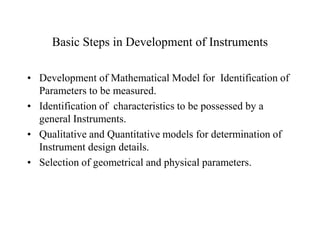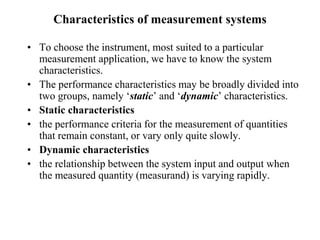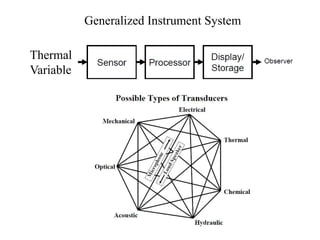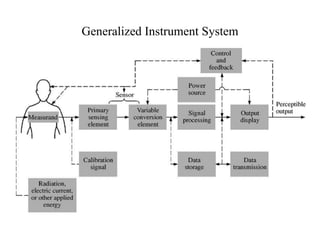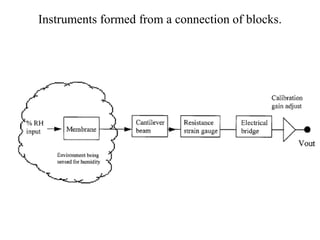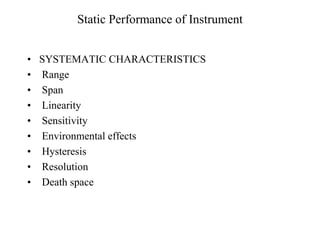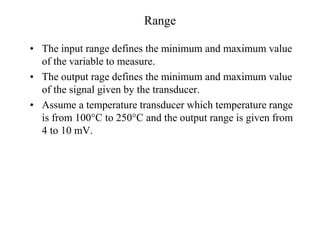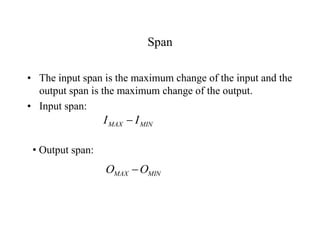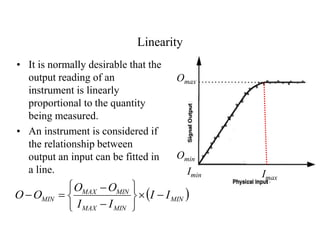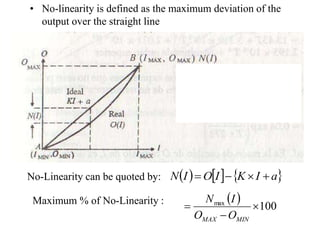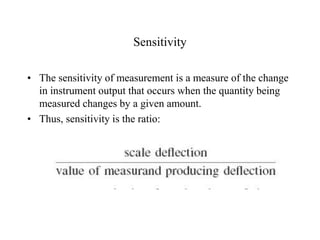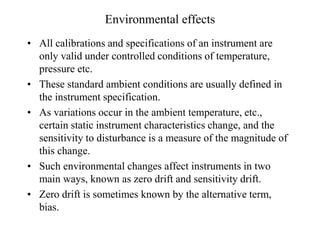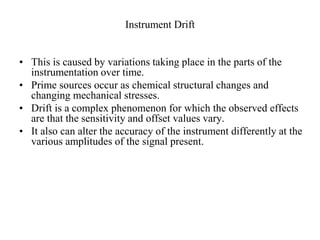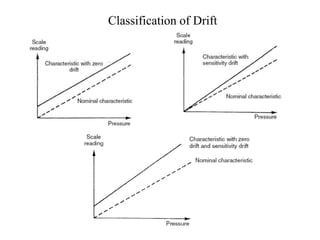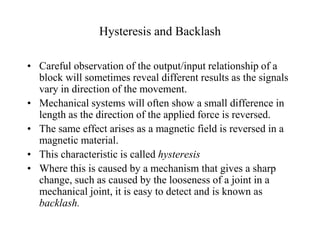1 de 16
Anúncio

### mel705-18.ppt

1. Characteristics of Instruments P M V Subbarao Professor Mechanical Engineering Department A Step Towards Design of Instruments….
2. Basic Steps in Development of Instruments • Development of Mathematical Model for Identification of Parameters to be measured. • Identification of characteristics to be possessed by a general Instruments. • Qualitative and Quantitative models for determination of Instrument design details. • Selection of geometrical and physical parameters.
3. Characteristics of measurement systems • To choose the instrument, most suited to a particular measurement application, we have to know the system characteristics. • The performance characteristics may be broadly divided into two groups, namely ‘static’ and ‘dynamic’ characteristics. • Static characteristics • the performance criteria for the measurement of quantities that remain constant, or vary only quite slowly. • Dynamic characteristics • the relationship between the system input and output when the measured quantity (measurand) is varying rapidly.
4. Generalized Instrument System Thermal Variable
5. Generalized Instrument System
6. Instruments formed from a connection of blocks.
7. Static Performance of Instrument • SYSTEMATIC CHARACTERISTICS • Range • Span • Linearity • Sensitivity • Environmental effects • Hysteresis • Resolution • Death space
8. Range • The input range defines the minimum and maximum value of the variable to measure. • The output rage defines the minimum and maximum value of the signal given by the transducer. • Assume a temperature transducer which temperature range is from 100°C to 250°C and the output range is given from 4 to 10 mV.
9. Span • The input span is the maximum change of the input and the output span is the maximum change of the output. • Input span: MIN MAX I I  • Output span: MIN MAX O O 
10. Linearity • It is normally desirable that the output reading of an instrument is linearly proportional to the quantity being measured. • An instrument is considered if the relationship between output an input can be fitted in a line. Imin Imax Omin Omax   MIN MIN MAX MIN MAX MIN I I I I O O O O            
11. • No-linearity is defined as the maximum deviation of the output over the straight line No-Linearity can be quoted by:       a I K I O I N     Maximum % of No-Linearity :   100 max    MIN MAX O O I N
12. Sensitivity • The sensitivity of measurement is a measure of the change in instrument output that occurs when the quantity being measured changes by a given amount. • Thus, sensitivity is the ratio:
13. Environmental effects • All calibrations and specifications of an instrument are only valid under controlled conditions of temperature, pressure etc. • These standard ambient conditions are usually defined in the instrument specification. • As variations occur in the ambient temperature, etc., certain static instrument characteristics change, and the sensitivity to disturbance is a measure of the magnitude of this change. • Such environmental changes affect instruments in two main ways, known as zero drift and sensitivity drift. • Zero drift is sometimes known by the alternative term, bias.
14. Instrument Drift • This is caused by variations taking place in the parts of the instrumentation over time. • Prime sources occur as chemical structural changes and changing mechanical stresses. • Drift is a complex phenomenon for which the observed effects are that the sensitivity and offset values vary. • It also can alter the accuracy of the instrument differently at the various amplitudes of the signal present.
15. Classification of Drift
16. Hysteresis and Backlash • Careful observation of the output/input relationship of a block will sometimes reveal different results as the signals vary in direction of the movement. • Mechanical systems will often show a small difference in length as the direction of the applied force is reversed. • The same effect arises as a magnetic field is reversed in a magnetic material. • This characteristic is called hysteresis • Where this is caused by a mechanism that gives a sharp change, such as caused by the looseness of a joint in a mechanical joint, it is easy to detect and is known as backlash.
Anúncio